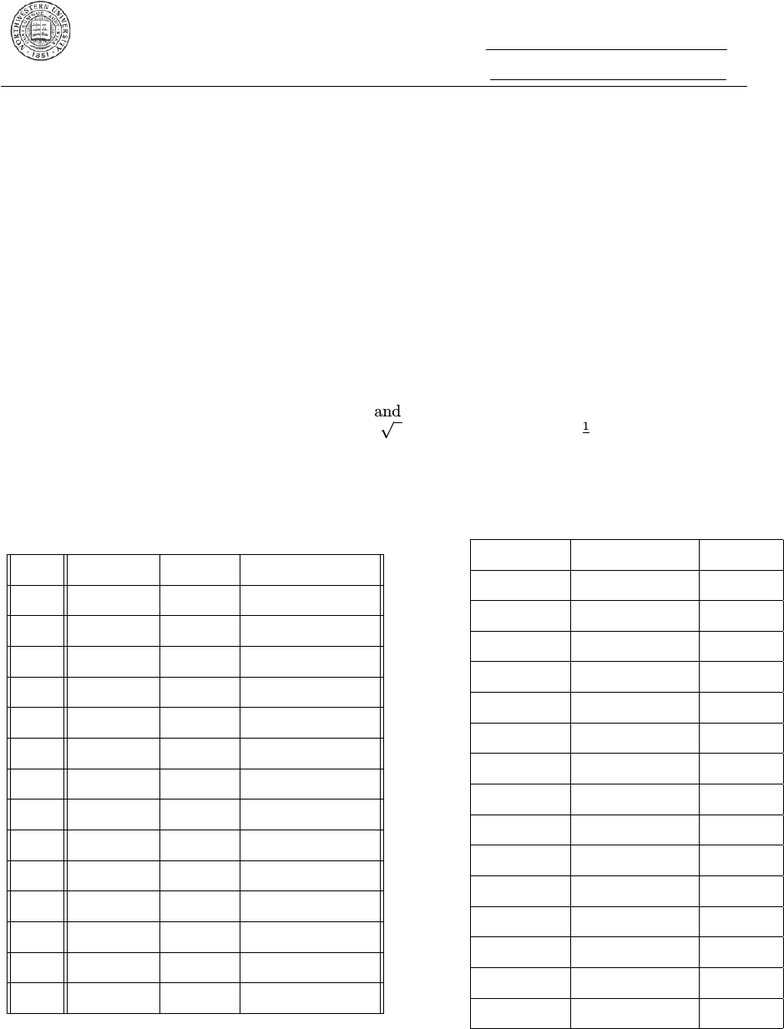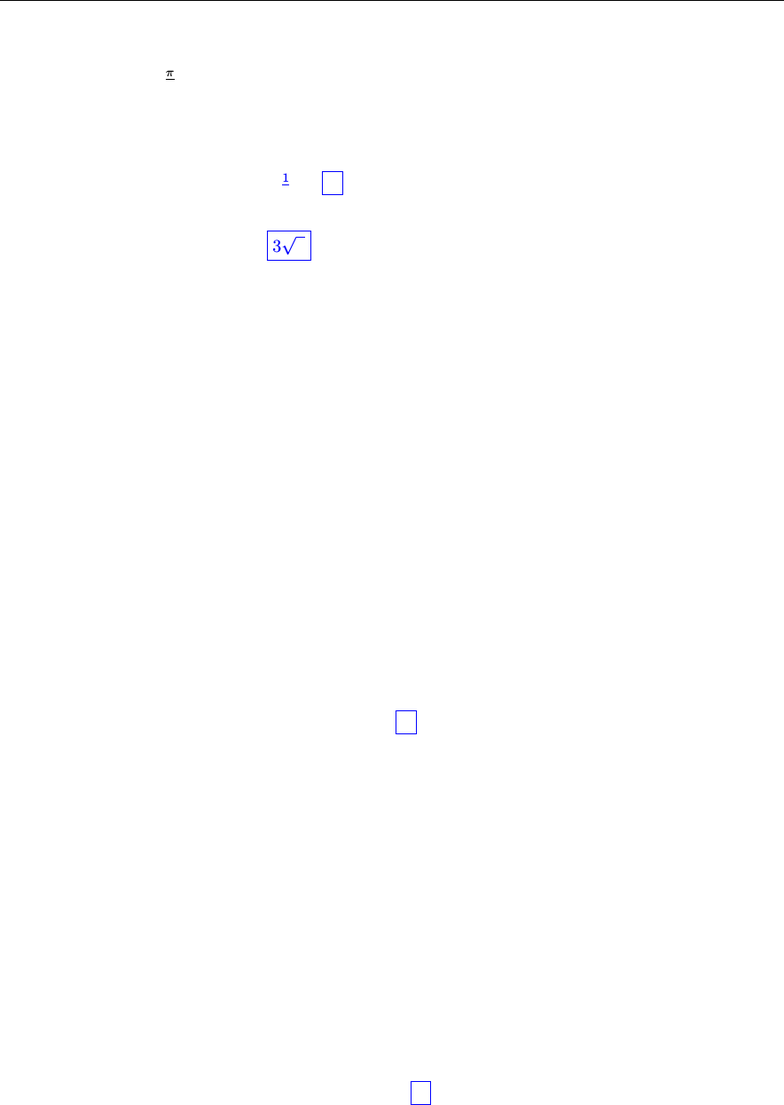# Final fall 2012 solutions

26 views17 pages
School
Department
Course
ProfessorNorthwestern University NetID:
ID #:
Math 230 Final Exam Solutions!
Fall Quarter 2012
Wednesday, December 12, 2012
Complete the front page!!!! Mark your section!! [3 points!]
Do not write your name on this exam!
Write your NetID and your Student ID Number in the spaces provided at
the top of this page.
Instructions: Show ALL of your work on the pages provided! Explain your reasoning using
complete sentences and correct grammar, spelling, and punctuation. All numerical answers MUST
be exact; e.g., you should write πinstead of 3.14...,2 instead of 1.414..., and 1
3instead of 0.3333...
Make sure that your ﬁnal answer is clearly indicated. This test has seventeen pages. Check to make
sure you have pages 1 - 17. Calculators, course notes, textbooks, etc. are NOT allowed. You have 2
hours! Good luck!
XSec. # Time Instructor
21 8:00 Garton
31 9:00 Bohmann
33 9:00 Tosatti
37 9:00 Yang
41 10:00 TiM
47 10:00 Tacy
49 10:00 Yang
57 11:00 Burslem
59 11:00 Garton
61 12:00 Burns
67 12:00 Tacy
71 1:00 Drummond-Cole
81 2:00 Burns
87 2:00 Drummond-Cole
Question Possible Points Score
Front Page 3
1 12
2 15
3 9
4 9
5 18
6 14
7 18
8 16
9 18
10 16
11 18
12 18
13 16
Total 200
Unlock document

This preview shows pages 1-3 of the document.
Unlock all 17 pages and 3 million more documents.

Already have an account? Log inMath 230 Final Exam Solutions! Fall Quarter 2012 Page 2 of 17
Question 1. Suppose that u,vare two vectors in R3such that kuk= 3, kvk= 2, and the angle
between them is θ=π
3.
(a) (3 points) Calculate u·vand ku×vk.
Answer:
u·v=kukkvkcos(θ) = 3(2)(1
2) = 3
ku×vk=kukkvksin(θ) = 33
(b) (5 points) Calculate (uv)·(u+ 2v).
Answer:
(uv)·(u+ 2v) = u·(u+ 2v)v·(u+ 2v)
=u·u+ 2u·vv·u2v·v
=kuk2+u·v2kvk2
= 9 + 3 8
= 4
(c) (4 points) Calculate (u+ 3v)·(3u×v).
Answer: Recall that u×vis orthogonal to both uand v, so
u·(u×v) = v·(u×v) = 0
Then,
(u+ 3v)·(3u×v) = 3u·(u×v) + 9v·(u×v)
= 0 + 0
= 0
Unlock document

This preview shows pages 1-3 of the document.
Unlock all 17 pages and 3 million more documents.

Already have an account? Log inMath 230 Final Exam Solutions! Fall Quarter 2012 Page 3 of 17
Question 2. For each of the following, calculate the limit or explain why the limit does not exist:
(a) (5 points) lim
(x,y)(0,0)
y3+ 5x2+ 5y2
x2+y2
Answer:
Method 1: Polar Coordinates Method 2: Sandwich Theorem
lim
r0
r3sin3(θ) + 5r2
r2= lim
r0rsin3(θ) + 5 = 5
(since 5 rrsin3(θ)5 + r)
=lim
(x,y)(0,0)
y3+ 5x2+ 5y2
x2+y2= 5
5− |y| ≤ y3+ 5x2+ 5y2
x2+y25 + |y|
lim
(x,y)(0,0) 5− |y|= lim
(x,y)(0,0) 5 + |y|= 5
=lim
(x,y)(0,0)
y3+ 5x2+ 5y2
x2+y2= 5
(b) (5 points) lim
(x,y,z)(0,0,0)
yz
x2+y2+z2.
Answer:
Method 1: Test Directions Method 2: Spherical Coordinates
Suppose y=z= 0 : lim
x0
0
x2= 0
Suppose x= 0, y =z: lim
y0
y2
2y2=1
26= 0
=lim
(x,y,z)(0,0,0)
yz
x2+y2+z2DNE
lim
ρ0
ρ2sin(θ) sin(ϕ) cos(ϕ)
ρ2= lim
ρ0sin(θ) sin(ϕ) cos(ϕ) :
Let θ=π
2,and ϕ= 0 : sin(θ) sin(ϕ) cos(ϕ) = 0
Let θ=π
2,and ϕ=π
4: sin(θ) sin(ϕ) cos(ϕ) = 1
2
Therefore, the limit depends on ϕ
=DNE
(c) (5 points) lim
(x,y)(0,0)
x4+y4
px4+y4+ 1 1
Answer:
lim
(x,y)(0,0)
x4+y4
px4+y4+ 1 1= lim
(x,y)(0,0)
x4+y4
px4+y4+ 1 1·px4+y4+ 1 + 1
px4+y4+ 1 + 1
= lim
(x,y)(0,0)
(x4+y4)(px4+y4+ 1 + 1)
(x4+y4+ 1) 1
= lim
(x,y)(0,0) px4+y4+ 1 + 1
= 2
Unlock document

This preview shows pages 1-3 of the document.
Unlock all 17 pages and 3 million more documents.

Already have an account? Log in

# Get OneClass Grade+

Unlimited access to all notes and study guides.

###### Grade+All Inclusive
\$10 USD/m
You will be charged \$120 USD upfront and auto renewed at the end of each cycle. You may cancel anytime under Payment Settings. For more information, see our Terms and Privacy.
Payments are encrypted using 256-bit SSL. Powered by Stripe.